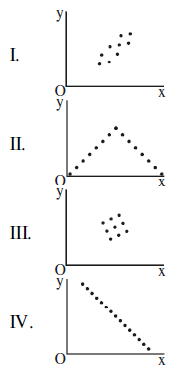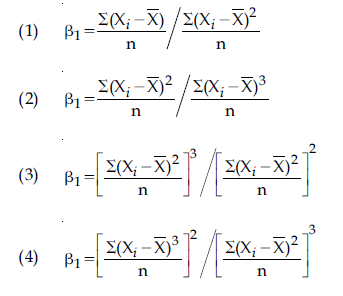# GEOGRAPHICAL TECHNIQUES PYQS JAN & NOV 2017 NTA UGC NET

GEOGRAPHICAL TECHNIQUES (CARTOGRAPHY & STATS) PYQS JAN & NOV 2017 NTA UGC NET

GEOGRAPHICAL TECHNIQUES PYQS JAN 2017 NTA UGC NET, GEOGRAPHICAL TECHNIQUES  NTA UGC NET JAN 2017,GEOGRAPHICAL TECHNIQUES  PYQS UGC NET NOV 2017, GEOGRAPHICAL TECHNIQUES  PYQS JULY 2017 NTA UGC NET PYQS GEOGRAPHY, GEOGRAPHICAL TECHNIQUES  PYQS UGC NET, PRACTICAL GEOGRAPHY PYQS NTA UGC NET, CARTOGRAPHY PYQS NTAUGC NET, STATS PYQS NTAUGC NET, GEOGRAPHY PYQS UGC NET,

(PDF) For download PDF file of 2017 UGC NET – go to the bottom of this post.

Q.1. Match List-I with List–II and select the correct answer from the codes given below: (UGC NET JAN 2017)

List-I

(Land use/Land cover types)

(I) Forests

(II) Cultivated areas

(III) Uncultivable land

(IV) Built up areas

List-II

(Conventional colour map symbols)

(A) Yellow

(B) Red

(C) Dark green

(D) Brown

Codes:

I II III IV

(1). C A D B

(2). D C B A

(3). A D B C

(4). C A B D

Q.2. Which of the following is a non-quantitative a real distribution map? (UGC NET JAN 2017)

(1) Choropleth

(2) Isopleth

(3) Multiple dots

(4) Choroschematic

Q.3. Cumulative frequency distribution is shown by: (UGC NET JAN 2017)

(1) Histogram

(2) Frequency curve

(3) Pie diagram

(4) Ogive

Q.4. Given below are two statements, one labelled as Assertion (A) and other labelled as Reason (R). Select your answer from the codes given below: (UGC NET JAN 2017)

Assertion (A): Maps that are usually referring various attributes’ information are called complex thematic maps

Reason (R): Complex Thematic Maps are used to show locations of earth’s different features and activities.

Codes:

(1) Both (A) and (R) are true and (R) is the correct explanation of (A)

(2) Both (A) and (R) are true, but (R) is not the correct explanation of (A)

(3) (A) is true but (R) is false

(4) (A) is false but (R) is true.

Q.5. Match List-I with List–II and select the correct answer from the codes given below: (UGC NET JAN 2017)

List- I (Distribution of Settlement)List-II (Country)

(A) Perfect Uniform

(B) Cluster

(3) C. Random

(4) D. Uniform

Codes:

I II III IV

(1) B C A D

(2) B C D A

(3) B A D C

(4) A B C D

Q.6. In order to study standard of living among the large, medium, small and marginal farmers of a region, which of the sampling technique would be more appropriate? (UGC NET JAN 2017)

(1) Random sampling

(2) Stratified Random sampling

(3) Opportunity sampling

(4) Multistage sampling

Q.7. Match List-I with List-II and select the correct answer from the codes given below: (UGC NET JAN 2017)

List 1 (Scatter Diagram)List 2 (The degree of simple rank correlation)

(A) ρ = −1 Perfect negative

(B) ρ = 0, no correlation

(C) ρ = < 1 Positive

(D) ρ = 1 Perfect positive

Codes:

I II III IV

(1) C D A B

(2) D C A B

(3) C D B A

(4) A B C D

Q.8. Which one of the following equations represents a true mathematical characteristic of mean? (UGC NET JAN 2017)

(1) ∑ (X- X̅)2 = minimum

(2) ∑ (X- X̅)2 = 0

(3) ∑ (X- X̅)2 = maximum

(4) ∑ (X- X̅)2 < ∑ (X- X̅)

Q.9. Given below are two statements, one labelled as Assertion (A) and the other labelled as Reason (R). Select your answer from the codes given below: (UGC NET JAN 2017)

Assertion (A): Area sampling by random numbers requires the area under study which should be gridded within two areas at right angle.

Reason (R): The intersections of row and column of the area will give the sample locations of observation in the area.

Codes:

(1) Both (A) and (R) are true and (R) is the correct explanation of (A).

(2) Both (A) and (R) are true, but (R) is not the correct explanation of (A).

(3) (A) is true, but (R) is false.

(4) (A) is false, but (R) is true.

Q.10. In linear regression equation, Y = a +bX, if mean of X and Y series are 23 and 94.5 respectively and slope gradient is 1.5, then what is the value of intercept a, in the distribution of (X, Y) series? (UGC NET JAN 2017)

(1) 50

(2) 55

(3 60

(4) 65

Q.11. Match List I with List II and select the correct answer from the codes given below: (UGC NET JAN 2017)

List –I (Value of Rn Statistics)

(I) < 0.5

(II) 0.75 – 1.25

(III) 1.75 – 2.00

(IV) 2.15

List-II (Pattern of Distribution)

(A) Perfect Uniform

(B) Tending to uniform

(C) Tending to random

(D) Tending to cluster

Codes:

I II III IV

(1). B A D C

(2). A B C D

(3). D C B A

(4). C D A B

Q.12. Which one of the following phenomenal relationships is depicted by an ergograph? (UGC NET NOV 2017)

(1) Temperature and absolute humidity

(2) Rainfall and runoff

(3) Crop production and rainfall

(4) Crop season and weather conditions

Q.13. Consider the following figures X and Y: (UGC NET NOV 2017)Which one of the following statements is correct?

(1) Figure X is small-scale map and distance between A and B is 40 metres.

(2) Figure Y is large-scale map and distance between C and D is 4 km.

(3) Figure X is large-scale map and figure Y is small-scale and distance between A and B and C and D are 40 metres and 20km, respectively.

(4) Figure X is large scale and figure Y is small-scale. The ground distance between A and B, and C and D are equal.

Q.14. Given below are two statements, one labelled as Assertion (A), and the other labelled as Reason (R). Select your answer from the code given below: (UGC NET NOV 2017)

Assertion (A): Dot map is especially useful when the values are distributed unevenly and sporadically.

Reason (R): Smaller the statistical unit, more accurate is the Dot map.

Code:

(1) Both (A) and (R) are true and (R) is the correct explanation of (A).

(2) Both (A) and (R) are true, but (R) is not a correct explanation of (A).

(3) (A) is true, but (R) is false.

(4) (A) is false, but (R) is true.

Q.15. Match List-I with the List –II and select the correct answer from the code given below: (UGC NET NOV 2017)

List-I

(Maps and Diagrams)

(A) Isopleth Maps

(B) Dot maps

(C) Scatter Diagram

(D) Star Diagram

List-II

(Aspect)

(i) Natural Grouping

(ii) Population Distribution

(iii) Wind Direction

(iv) Changes over space which are relatively gradual

Code:

(A) (B) (C) (D)

(1) (iv) (ii) (i) (iii)

(2) (iv) (i) (iii) (ii)

(3) (i) (ii) (iii) (iv)

(4) (ii) (iii) (iv) (i)

Q.16. Consider the following line symbols: (UGC NET NOV 2017)

(A) Isobars

(B) Contours

(C) Hachures

(D) Isochrones

Which of the above depicts relief?

Select the correct answer using depicts relief? Select the correct answer using the code given below:

(1) (a) and (d)

(2) (a) and (b)

(3) (b) and (c)

(4) (b) and (d)

Q.17. Which one of the following terms is correct for calculation of skewness coefficient β1? (UGC NET NOV 2017)Q.18. Given below are two statements, one labelled as Assertion (A), and the other labelled as Reason (R). Select your answer from the code given below: (UGC NET NOV 2017)

Assertion (A): Standard Deviation of a series is the measure of its variation.

Reason (R): Standard Deviation is needed to compare the variation between two or more series.

Code:

(1) Both (A) and (R) are true and (R) is the correct explanation of (A).

(2) Both (A) and (R) are true, but (R) is not a correct explanation of (A).

(3) (A) is true, but (R) is false.

(4) (A) is false, but (R) is true.

Q.19. Which one of the following terms is used to measure the extreme peak in a normal distribution? (UGC NET NOV 2017)

(1) Skewed

(2) Mesokurtic

(3) Leptokurtic

(4) Platykurtic